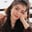Related Tags

seaborn

# What are distplots in Seaborn?Fouzia Bashir

## Overview

We use a displot (also known as a distribution plot) to represent data in histogram form. It is a univariant set of collected data, which means the data distribution of one variable will be shown against another variable.

In Python, we use the Seaborn library with Matplotlib for data visualization. It provides an interface that allows users to present data in various descriptive and eye-catching graphics in statistical form. Seaborn will enable us to deliver data using the types below:

1. displot
2. jointplot
3. pairplot
4. rug plot

Now let’s dive deeper.

In Seaborn, we use the seaborn.displot() function to display one data variable's distribution against the other variable as density distribution.

### Example

The function seaborn.displot() takes data variable and color as arguments and returns data in distribution form.

# import libraries
import numpy as np
import seaborn as sns
import matplotlib.pyplot as plt
# code to generate random numbers
data = np.random.randn(500)
# plot distplot
sns.displot(data, color="red")
plt.title("Data Distribution")
plt.savefig('output/graph.png')

### Explanation

• Lines 2–4: We'll import the numpy, seaborn, and matplotlib libraries in the program.
• Line 6: We'll use the np.random.randn(500) to generate an array of random numbers. In this line, it will create 500 random values.
• Line 8: We'll use the seab.displot(data) function to generate a distribution plot. We use the seab.displot(data) function to plot a distribution plot of data in the form of a histogram.
• Line 9: We'll use the plt.title() function to set the graph title to "Data Distribution".
• Line 10: We'll use the plt.savefig() function to save the graph generated above as graph.png in the output directory.

RELATED TAGS

seaborn

CONTRIBUTORFouzia Bashir
RELATED COURSES

View all Courses

Keep Exploring

Learn in-demand tech skills in half the time